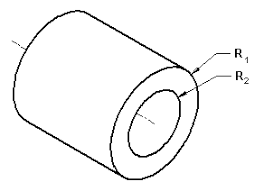## How to Calculate and Solve for Mass of Cylindrical Shaft | Material SelectionThe image above represents mass of cylindrical shaft.

To compute for mass of cylindrical shaft, five essential parameters are needed and these parameters are Factor of Safety (N), Twisting Moment (M), Length of Shaft (L), Density (ρ) and Shear Stress at Fracture (τf).

The formula for calculating mass of cylindrical shaft:

m = (2NM)2/31/3L)(ρ/τf2/3)

Where:

m = Mass of Cylindrical Shaft
N = Factor of Safety
M = Twisting Moment
L = Length of Shaft
ρ = Density
τf = Shear Stress at Fracture

Let’s solve an example;
Find the mass of cylindrical shaft when the factor of safety is 4, the twisting moment is 2, the length of shaft is 7, the density is 6 and the shear stress at fracture is 10.

This implies that;

N = Factor of Safety = 4
M = Twisting Moment = 2
L = Length of Shaft = 7
ρ = Density = 6
τf = Shear Stress at Fracture = 10

m = (2NM)2/31/3L)(ρ/τf2/3)
m = (2(4)(2))2/3 (π1/3(7)) (6/102/3)
m = (16)2/3 ((1.46)(7)) (6/4.64)
m = (6.349) (10.25) (1.29)
m = 84.14

Therefore, the mass of cylindrical shaft is 84.14 kg.